PART – 2: Text Processing and String Manipulation

1.1 Types   1.2 Array Declaration and Initialization   1.3 Traversal of the Array

2.1 Pointer Arithmetic     2.2 Array and Pointer

3.1 Types    3.2 Function Definition    3.3 Function Call        3.4 Function Declaration/ Prototype       3.5 Parameter Passing in C      3.6 return keyword in C      3.7 Interface and Implementation      3.8 Passing Array to a Function       3.9 Recursion

4.1 Declaration and Initialization of String      4.2 Read and Display Strings in C      4.3 Pointers v/s String      4.4 Built-in String Functions        4.5 String operations using User defined functions

Chapter 5: Solution for the Problem Text Processing and String Manipulation

-------------------------------------------------------------------------

## Chapter 1: Arrays

When solving problems, it is important to visualize the data related to the problem. Sometimes the data consist of just a single number (as we discussed in part-1, primitive types).  At other times, the data may be a coordinate in a plane that can be represented as a pair of numbers, with one number representing the x-coordinate and the other number representing the y- coordinate. There are also times when we want to work with a set of similar data values, but we do not want to give each value a separate name. For example, we want to read marks of 1000 students and perform several computations. To store marks of thousand students, we do not want to use 1000 locations with 1000 names. To store group of values using a single identifier, we use a data structure called an Array.

“Array consists of a group of elements of same data type. We can think it as collection of variables of similar data type.”

Characteristics/Properties of arrays:

Non-primary data type

Memory allocation is contiguous in nature

Elements need not be unique.

Demands same /homogenous types of elements

Elements are accessed using index/subscript

Index or subscript starts from 0

Memory is allocated at compile time.

Size of the array is fixed at compile time. Cannot change the size at runtime.

Accessing elements of the array outside the bound can have undefined behaviour at runtime.

### 1.1 Types

Can be broadly classified into two categories:

Fixed Length Array

Variable Length Array - Not supported by older few C standards. We are not discussing as a part of this unit.

### 1.2 Array Declaration and Initialization

Declaration:

data_type array_name[size]; // Declaration syntax

Consider int a1;

Declaration allocates number of bytes in a contiguous manner based on the size specified. All these memory locations are filled with undefined values. If the starting address is 0x5000 and if the size of integer is 4 bytes, refer the diagrams below to understand this declaration clearly. Number of bytes allocated = size specified*size of integer i.e, 10*4.

printf("size of array is %d\n", sizeof(a1)); // 40 bytes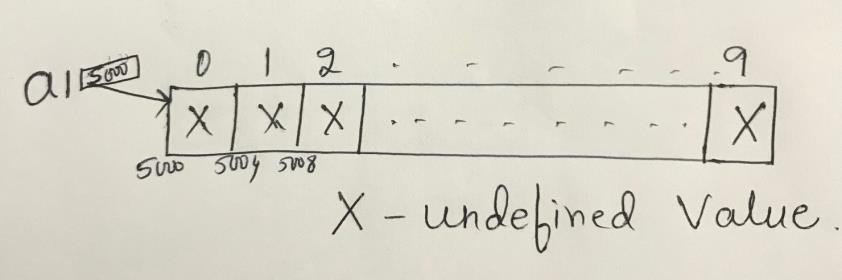Address of the first element is called the Base address of the array. Address of ith element of the array can be found using formula:

Address of ith element = Base address + (size of each element * i)

Examples of declarations:

char ch;

double d;

float f;

Initialization:

Consider int a2[ ] = {44,33,10,25,78,33,89};

Above initialization does not specify the size of the array. Size of the array is based on the number of elements stored in it and the size of type of elements stored. So, the above array occupies 7*4 = 28 bytes of memory in a contiguous manner.

printf("size of array is %d\n", sizeof(a2)); //28 bytes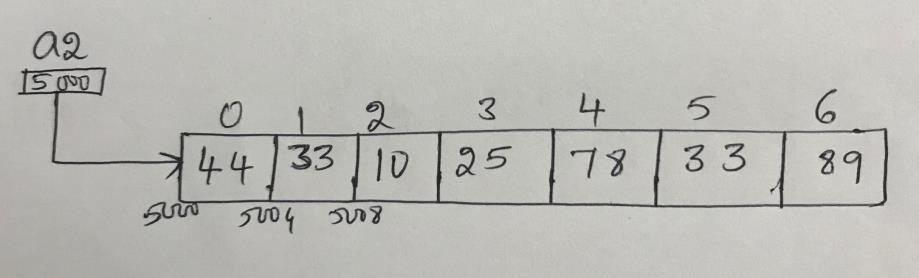Partial Initialization:

Consider int a3 = {44,33,10,25,78,33,89};

Size of the array is specified. But only few elements are stored. Now, the size of the array is 10*4 = 40 bytes of memory in a contiguous manner. All uninitialized memory locations in the array are filled with default value 0.

printf("size of array is %d\n", sizeof(a3)); //40 bytes

printf("%p %p\n",a3, &a3); // Must be different.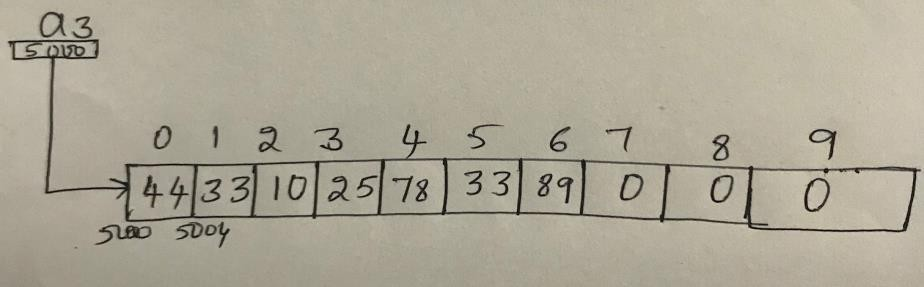### 1.3 Traversal of the Array

Accessing each element of the array is known as traversing the array.

Consider int a1;

How do you access the 5th  element of the array? a1

How do you display each element of the array?

int i;

for(i = 0; i<10;i++)

printf("%d  \t",a1[i]); //Using the index operator [ ].

Above code prints undefined values as a1 is just declared.

Now Consider int a2[ ] = {12,22,44,14,77,911};

int i;

for(i = 0; i<10;i++)

printf("%d  \t",a2[i]); //Using the index operator [ ].

Above code prints initialized values when i is between 0 to 5. When i becomes 6, a2 is outside bound as the size of a2 is fixed at compile time and it is 6*4(size of int is implementation specific) = 24 bytes. Anytime accessing elements outside the array bound is an undefined behaviour.

Example Code 1:

Let us consider the program to read 10 elements from the user and display those 10 elements. #include<stdio.h>

int main()

{ int arr; int n;

printf("enter the number of elements\n");

scanf("%d",&n);

printf("enter %d elements\n",n);

int i = 0;

while(i<n)

{ scanf("%d",&arr[i]);

i++;

printf("entered elements are\n");

i = 0;

while(i<n)

{ printf("%d\n",arr[i]); i++;

return 0;}

Example Code 2:

Let us consider the program to find the sum of the elements of an array. int arr[] = {-1,4,3,1,7};

int i; int sum = 0;

int n = sizeof(arr)/sizeof(arr); for(i = 0; i<n; i++)

{ sum += arr[i]; }

printf("sum of elements of the array is %d\n", sum);

// Think about writing a function to find the sum of elements of the array.

Few questions to think about above codes?

Should I use while only? for and while are same in C except for syntax.

Is there any clear demarcation between interface and implementation? No. Everything

is in the same file i.e. client . Try to separate these two

Can I use arr[n] while declaration? Variable length array

Can we write read and display user defined functions to do  the same? Yes. To do this, we should understand functions and how to pass array to a function. Refer to Chapter

– 3 and specifically 3.8 for more details

## Chapter 2: Pointers

Pointer is a variable which contains the address. Pointers can be used to access and manipulate data stored in memory.

Pointer of particular type can point to address any value of that particular type.

Consider,

int *p; // p can point to anything where integer is stored. int* is the type. Not just int.

int a = 100;

printf("a is %d and *p is %d", *p);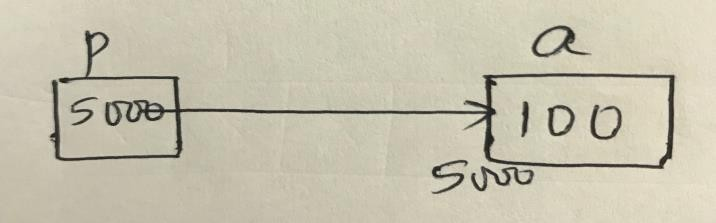Now, int arr[] ={12,44,22,33,55};

int  *p1 = arr; // same as int *p1; p1 = arr;

// same as int *p1; p1 = &arr;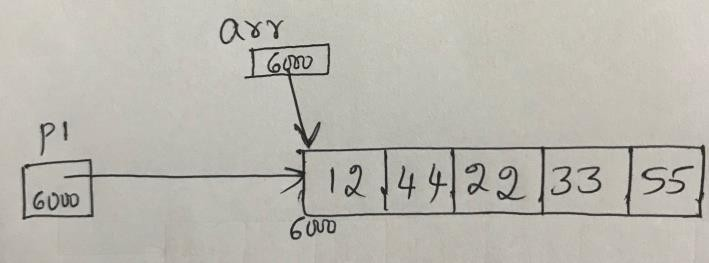int arr2;

arra2 = arr; // Arrays are assignment incompatible. Compile time Error

### 2.1 Pointer Arithmetic

Below arithmetic operations are allowed on pointers

Add an int to a pointer

Subtract an int from a pointer

Difference of two pointers when they point to the same array.

Integer is not same as pointer. We get warning when we try to compile the code where in integer is stored in variable of int* type.

int arr = {12,33,44};

int *p2 = arr;

printf("before increment %p %d\n",p2, *p2); // 12

p2++; //same as p2 = p2+1 // This means 5000+sizeof(every element)*1 if 500 is the base address

//increment the pointer by 1. p2 is now pointing to next location.

printf("after increment %p %d\n",p2, *p2); // 33

Let us concentrate on Array Traversal using pointers

int arr[] ={12,44,22,33,55};

int *p3 = arr; int i;

Write diagrams to understand this clearly.

Version 1: Index operator can be applied on pointer. Array notation

for(i = 0;i<5;i++)

printf("%d \t",p3[i]); //  12 44 22 33 55

// every iteration added i to p3 . p3 not modified

Version 2: Using pointer notation

for(i = 0;i<5;i++)

printf("%d \t",*(p3+i)); //  12 44 22 33 55

// every iteration i value is added to p3 and content at that address is printed. p3 not modified

Version 3:

for(i = 0;i<5;i++)

printf("%d \t",*p3++); //  12 44 22 33 55

// Use p3, then increment, every iteration p3 is incremented.

Version 4: undefined behaviour if you try to access outside bound

for(i = 0;i<5;i++)

printf("%d \t",*++p3); //  44 22 33 55 undefined value

// every iteration p3 is incremented.

Version 5:

for(i = 0;i<5;i++)

printf("%d \t",(*p3)++); //  13 14 15 16 17

// every iteration value at p3 is used and then incremented.

Version 6:

for(i = 0;i<5;i++,p3++)

printf("%d \t",*p3); //  13 14 15 16 17

// every iteration value at p3 is used and then p3 is incremented.

Version 7: p3 and arr has same base address of the array stored in it. But array is a constant pointer. It cannot point to anything in the world.

for(i = 0;i<5;i++)

printf("%d \t", *arr++); // Compile Time Error

### 2.2 Array and Pointer

An array during compile time is an actual array but degenerates to a constant pointer during run time. Refer to Section 3.8 for more details

Size of the array returns the number of bytes occupied by the array. But the size of pointer is always constant in that particular system.

Examples: int *p1; float *f1 ; char *c1;

printf("%d %d %d ",sizeof(p1),sizeof(f1),sizeof(c1)); // Same value for all

int a[] = {22,11,44,5};

int *p = a;

a++;// Error

p++; // Fine

p = 222; // Undefined Behaviour

a = 222 ; // Fine

If variable i is used in loop for the traversal, a[i], *(a+i), p[i], *(p+i), i[a], i[p] are all same.

## Chapter 3: Functions

Functions break large computing tasks into smaller ones and enable people to build on what others have done instead of starting from scratch. In programming, Function is a subprogram to carry out a specific task.

A function is a self-contained block of code that performs a particular task. Once the function is designed, it can be treated as a black box. The inner details of operation are invisible to rest of the program.

Benefits of using functions.

Reduced Coding Time – Code Reusability

Modularity.

Reduced Debugging Time

Sharing

Maintainability

### 3.1 Types

C functions can be broadly classified into two categories:

Library Functions

⦁ Functions which are defined by C library. Examples include printf(), scanf(), strcat() etc. We                just need to include appropriate header files to use these functions. These are already declared                and defined in C libraries.

User-defined Functions

⦁ Functions which are defined by the developer at the time of writing program.

⦁ Developer can make changes in the implementation as and when he/she wants.

In order to make use of user defined functions, we need to understand three key terms associated with functions: Function Definition, Function call and Function Declaration.

### 3.2 Function Definition

Each function definition has the form

return_type function_name(paratmeters) // parameters optional

{

// declarations and statements

}

A function definition should have a return type. The return type must match with what that function returns at the end of the function execution. If the function is not designed to return anything, then the type must be mentioned as void. Like variables, function name is also an identifier and hence must follow the rules of an identifier. Parameters list is optional. If more than one parameter, it must be comma separated. Each parameter is declared with its type and parameters receive the data sent during the function call. If function has parameters or not, but  the parentheses is must. Block of statements inside the function or body of the function must be inside { and }. There is no indentation requirement as far as the syntax of C is considered. For readability purpose, it is a good practice to indent the body of the function.

Function definitions can occur in any order in the source file and the source program can be split into multiple files.

int sum(int a, int b)

{

return a+b;

}

int decrement(int y)

{

return y-1;

}

void disp_hello() // This function doesn’t return anything

{

printf("Hello Friends\n");

}

double use_pow(int x)

{

return pow(x,3); // using pow() function is not good practice.

}

int fun1(int a, int) // Error in function definition.

{

}

int fun2(int a, b) // Invalid Again.

{}

### 3.3 Function Call

Function-name(list of arguments); // semicolon compulsory

// Arguments depends on the number of parameters in the function definition

A function can be called by using function name followed by list of arguments (if any) enclosed in parentheses. The function which calls other function is known to be Caller and the function which is getting called is known to be the Callee. The arguments must match the parameters in the function definition in it’s type, order and number. Multiple arguments must be separated by comma. Arguments can be any expression in C. Need not be always just variables. A function call is an expression. When a function is called, Activation record is created. Activation record is another name for Stack Frame. It is composed of:

Local variables of the callee

Location to store return value

Parameters of the callee

Temporary variables

The order in which the arguments are evaluated in a function call is not defined and is determined by the calling convention(out of the scope of this notes) used by the compiler. It is left to the compiler Writer. Always arguments are copied to the corresponding parameters. Then the control is transferred to the called function. Body of the function gets executed. When all the statements are executed, callee returns to the caller. OR when there is return statement, the expression of return is evaluated and then callee returns to the caller. If the return type is void, function must not have return statement inside the function.

#include<stdio.h>

int main()

{

int x = 100; int y = 10;

disp_hello();

double ans = use_pow(x);

printf("ans is %lf\n",ans);

printf("power : %lf\n", use_power(5));

return 0;

}

### 3.4 Function Declaration/ Prototype

All functions must be declared before they are invoked. The function declaration is as follows.

return_type  Function_name (parameters list); // semicolon compulsory

The parameters list must be separated by commas. The parameter names do not need to be the same in declaration and the function definition. The types must match the type of parameters  in the function definition in number and order. Use of identifiers in the declaration is optional. When the declared types do not match with the types in the function definition, compiler will produce an error.

Interface and Implementation

The function declaration is the interface. The function Definition is the implementation. Interface tells us what the function expects. It does not tell us how the function works.

If we change the function definition, the user or the client of the function is not affected.

Refer to 3.7 for details

### 3.5 Parameter Passing in C

Parameter passing is always by Value in C

Argument is copied to the corresponding parameter. The parameter is not copied back to the argument. It is possible to copy back the parameter to argument only if the argument is l- value. Argument is not affected if we change the parameter inside a function.

Version 1:

void fun1(int a1); // declaration

void main(){

int a1 = 100;

printf("before function call a1 is %d\n", a1); // a1 is 100

fun1(a1); // call

printf("after function call a1 is %d\n", a1); // a1 is  100

}

void fun1(int a1)

{

printf("a1 in fun1 before changing %d\n", a1); //100

a1 = 200;

printf("a1 in fun1 after changing %d\n", a1); //200

}

a1 has not changed in main function. The parameter a1 in fun1 is a copy of a1 from main function. Refer to the below diagram to understand activation record creation and deletion to know this program output in detail.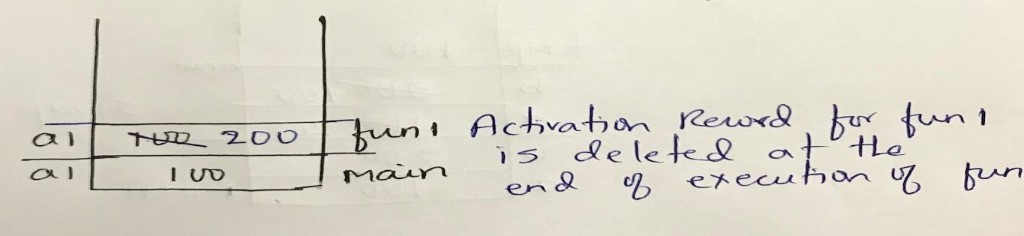If yes, then how library functions work? Example: scanf, strcpy, strcncpy and so on.

Answer is No. It is possible to change the argument if we pass l-value

Version 2:

void fun1(int *a1); int main(){

int a1 = 100;

printf("before function call a1 is %d\n", a1); // 100

fun1(&a1); // call

printf("after function call a1 is %d\n", a1); // 200

}

void fun1(int *a1)

{

printf("*a1 in fun1 before changing %d\n", *a1); //100

*a1 = 200;

printf("*a1 in fun1 after changing %d\n", *a1); //200

}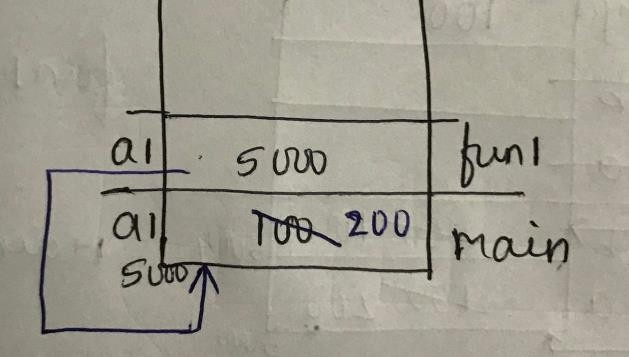Version 3:

void fun1(int *a1); int main(){

int a1 = 100;

printf("before function call a1 is %d\n", a1); // 100

fun1(&a1); // call

printf("after function call a1 is %d\n", a1); // 100

}

void fun1(int *a1)

{ int b = 200;

printf("*a1 in fun1 before changing %d\n", *a1); //100  a1 = &b;

printf("*a1 in fun1 after changing %d\n", *a1); //200

}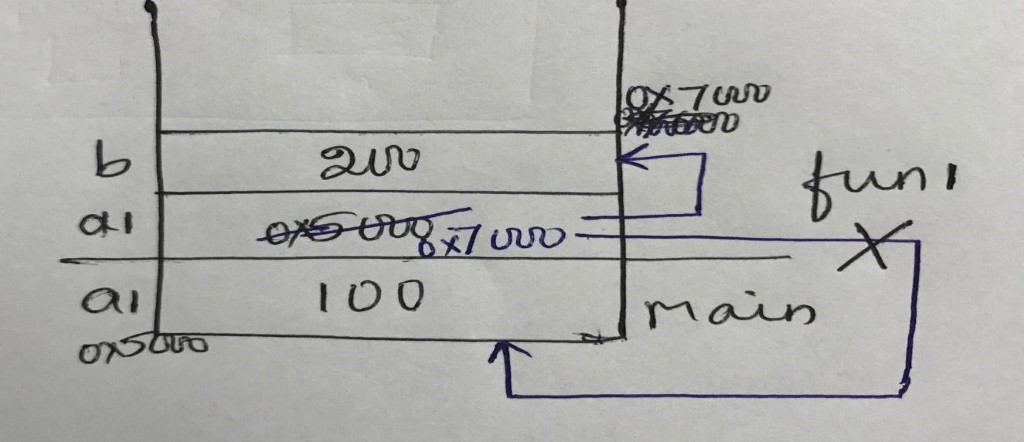Shall we write the function to swap two numbers and test this function?

Version – 1: Is this right version?

void swap(int a, int b)

{ int temp = a; a = b; b = temp;

int main()

{ int a = 100; int b = 200;

printf("before call a is %d and b is %d\n", a, b); // a is 100 and b is 200 swap(a, b);

printf("after call a is %d and b is %d\n", a, b); // a is 100 and b is 200

}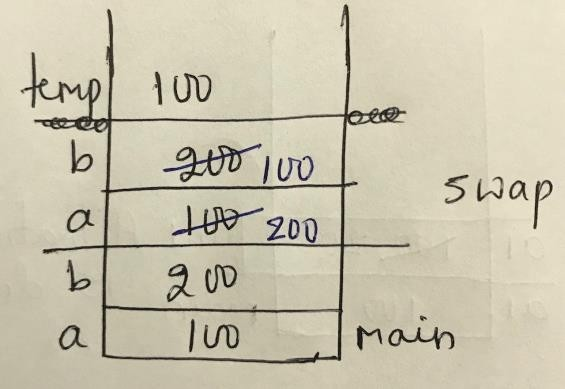Version 2:

void swap(int *a, int *b)

{ int temp = *a; *a = *b; *b = temp; } int main()

{ int a = 100; int b = 200;

printf("before call a is %d and b is %d\n", a, b); // a is 100 and b is 200 swap(&a, &b);

printf("after call a is %d and b is %d\n", a, b); // a is 100 and b is 200

}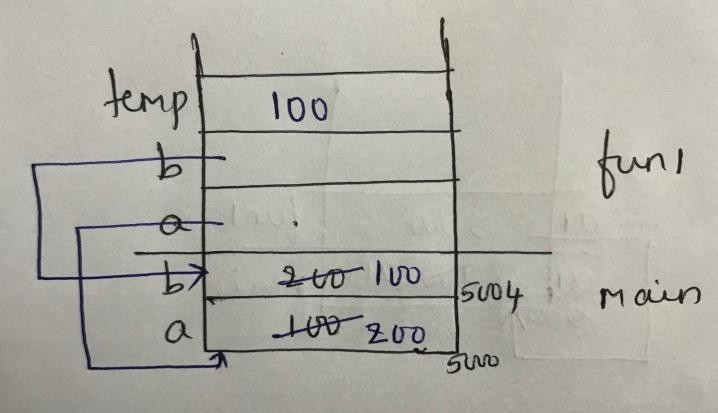3.6 return keyword in C

Function must do what it is supposed to do. It should not do something extra. For Example, refer to the below code.

{

return a+b;

}

The function add() is used to add two numbers. It should not print the sum inside the

function. We cannot use this kind of functions repeatedly if it does something extra than what is required. Here comes the usage of return keyword inside a function. Syntax: return expression;

In ‘C’, function returns a single value. The expression of return is evaluated and copied to the temporary location by the called function. This temporary location does not have any name. If the return type of the function is not same as the expression of return in the function definition, the expression is cast to the return type before copying to the temporary location. The calling function will pick up the value from this temporary location and use it.

void f1(int);

void f2(int*);

void f3(int);

void f4(int*);

int* f5(int* );

int* f6();

int main()

{ int x = 100;

f1(x);

printf("x is %d\n", x); // 100

double y = 6.5;

f1(y); // observe what happens when double value is passed as

//argument to integer parameter?

printf("y is %lf\n", y);              // 6.500000

int *p = &x; // pointer variable

f2(p);

printf("x is %d and *p is %d\n", x, *p); // 100 100

f3(*p);

printf("x is %d and *p is %d\n", x, *p); // 100 100

f4(p);

printf("x is %d and *p is %d\n", x, *p, p);       // 10 10

int z= 10;

p = f5(&z);

printf("z is %d  and %d\n", *p, z); // 10  10

p = f6();

printf("*p is %d \n", *p);

}

void f1(int x)

{

x = 20;

}

void f2(int* q)

{

int temp = 200;

q = &temp;

}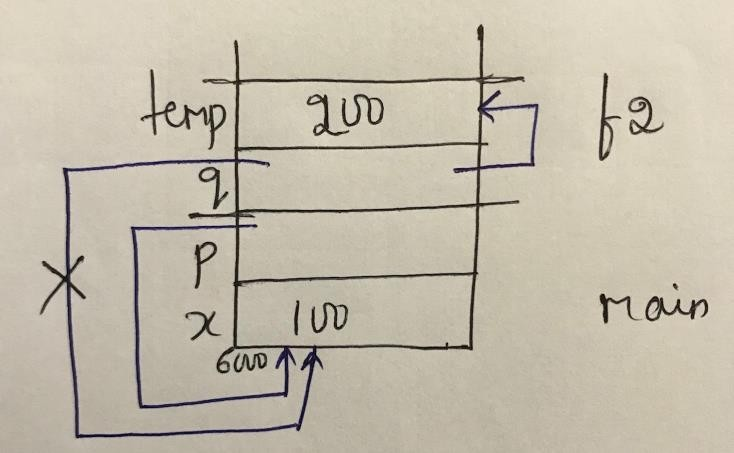void f3(int t)

{

t = 200;

}

void f4(int* q)

{

int temp = 200;

*q = temp;

}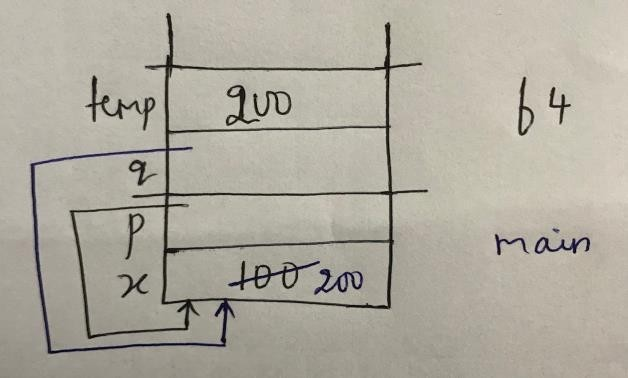int* f5(int* x)

{

return x;

}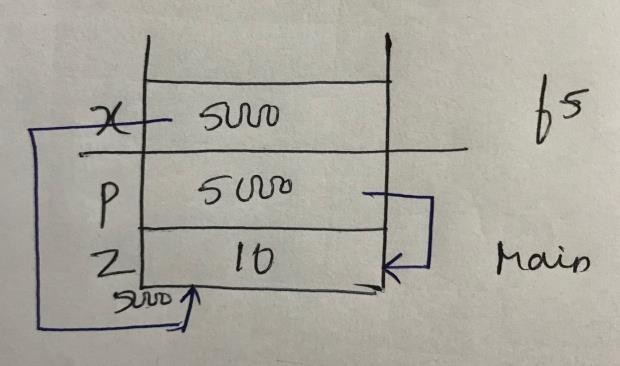int* f6()

{

int a = 22; // We should never return a pointer to a local variable

return &a; // Compile time Error

}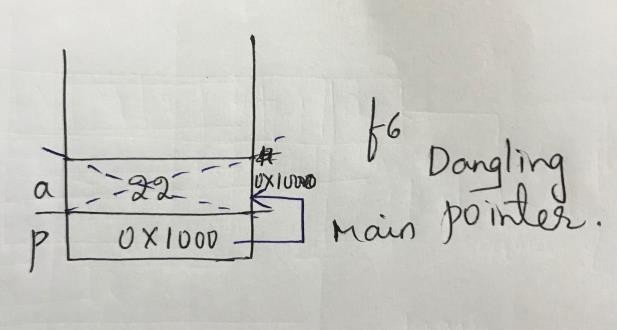When there is function call to f6, Activation Record gets created for that and deleted when the function returns. p points to a variable which is not available after the function execution. This problem is known as Dangling Pointer. The pointer is available. But the location it is not available which is pointed by pointer. Applying dereferencing operator(*) on a dangling pointer is always undefined behaviour.

### 3.7 Interface and Implementation

Interface means Function declaration. Implementation means Function definition. Interface tells us what the function requires while calling. It does not tell us anything about how that function works. Implementation always refers to the body of the function. If any change in the body of the function, it does not affect outside. The one who is using the function, need not worry about the change in implementation.

Let us say, requirement is to check whether a given number is a palindrome or not.

Version 1:

#include<stdio.h>

#include “palin.h”

int main()

{ int n;

printf("Enter the number\n");

scanf("%d", &n);

int rev = 0; int number = n;

while(n>0) // can be just while(n). When n is 0, loop terminates.

{ rev = rev * 10 + n % 10;

n /= 10;

}

if(number == rev)

printf("%d is palindrome\n",number);

else

printf("%d is not palindrome\n",number);

}

Version 2:

Now we will write the function to check whether the given number is palindrome or not. #include<stdio.h>

int is_palin(int); // interface

int main()

{ int n;

printf("Enter the number\n");

scanf("%d", &n);

if(is_palin(n))

{

printf("%d is a palindrome\n", n);

}

else

{

printf("%d is not a palindrome\n", n);

}

}

int is_palin(int n) // implementation

{ int rev = 0; int number = n;

while(n>0)

{ rev = rev * 10 + n % 10; n /= 10;

}

return number == rev;

}

You might want to write reverse function which reverses the given number and the return  value of that can be used for checking inside the is_palin function. Try it!

Version 3:

Differentiate between client code, server code and the header files.

Declarations of functions in palin.h

int is_palin(int n);

Then we write the client code [where the execution starts – main() in C ] as below. client.c

contains the below code.

#include<stdio.h>

#include “palin.h” // interface

int main()

{ int n;

printf("Enter the number\n");

scanf("%d", &n);

if(is_palin(n))

{

printf("%d is a palindrome\n", n);

}

else

{

printf("%d is not a palindrome\n", n);

}

}

Definitions of User defined functions are saved in palin.c

int is_palin(int n) // implementation

{ int rev = 0;

int number = n;

while(n>0)

{ rev = rev * 10 + n % 10; n /= 10;

}

return number == rev;

}

Commands to execute above codes is as below.

gcc -c client.c // this creates client.o

gcc -c palin.c //  this creates palin.o

gcc client.o palin.o // this is for linking. We get the loadable image a.exe or a.out

a.exe  or  ./a.out // press enter to see the result or output

If I make some changes in the implementation file i.e., palin.c, I need to compile only that. Then link palin.o and client.o to get the executable.

If there are one or two implementation files, the changed files can be recompiled and relinked

easily. But, if you have many implementation files and you have made modifications to some of these, you need to remember which all files you need to compile again. Or Compile all files and link all object files again. This is waste of time. Rather, we have a facility called make which completes this requirement in an easier way.

Usage of make command

make is Unix utility that is designed to start execution of a makefile. A makefile is a special file, containing shell commands, that you create and name it with .mk extension preferably. While in the directory containing this makefile, you will type make and the commands in the makefile will be executed.

A makefile that works well in one shell may not execute properly in another shell.

Because it must be written for the shell which will process the makefile.

The makefile contains a list of rules. These rules tell the system what commands you want to be executed. These rules are commands to compile(or recompile) a series of files. The rules, which must begin in column 1, are specified in two types of lines. The first line is called a Dependency line and the subsequent line(s) are called Action lines. The action line(s) must be indented with a tab.

The dependency line is made of two parts. The first part (before the colon) are Target files and the second part (after the colon) are called Source files. It is called a dependency line because the first part depends on the second part. Multiple target files must be separated by a space. Multiple source files must also be separated by a space.

Make reads the makefile and creates a dependency tree and takes whatever action is necessary. It will not necessarily do all the rules in the makefile as all dependencies may not need updated. It will rebuild target files if they are missing or older than the dependency files. It keeps track of the recent time files (normally object files) were updated and only updates those files which are required (ones containing changes) to keep the sourcefile up-to-date. If you have a large program with many source and/or header files, when you change a file on which others depend, you must recompile all the dependent files. Without a makefile, this is an extremely time-consuming task.

Command to execute on Ubuntu:

make   -f  filename.mk // -f to read file as a make file

If you have installed gcc using mingw package, go to mingw/bin folder in command prompt and type as below.

mingw-get install mingw32-make // press enter

Command to execute on Windows

mingw32-make  -f  filename.mk // -f to read file as a make file

Corresponding Make file for palindrome checking code is as below.

a.out : client.o palin.o

gcc client.o palin.o client.o : client.c palin.h

gcc -c client.c palin.o : palin.c palin.h

gcc -c palin.c

### 3.8 Passing Array to a Function

When array is passed as an argument to a function, arguments are copied to parameters of the function and parameters are always pointers. Array degenerates to a pointer at runtime. All the operations that are valid for pointer will be applicable for array too in the body of the function. Function call happens always at run time.

#include<stdio.h> int main()

{ int arr; int n;

printf("Enter the number of elements you want to store\n");

scanf("%d",&n);

printf("enter %d elements\n",n);

printf("entered elements are\n");

display(arr,n);

}

void read(int arr[],int n) // arr is an array. But it becomes pointer at runtime

{ // check the sizeof(arr). It is same as size of pointer int i = 0;

while(i<n)

{ scanf("%d",&arr[i]);

i++;

}

}

void display(int arr[],int n)

{

int i = 0; while(i<n)

{ printf("%d",arr[i]);

i++;

}

}

So, use pointer variable in the parameters of the function definition when array is passed as the argument.

void read(int *arr , int n)

{

int i = 0;

while(i<n)

{ scanf("%d",&arr[i]);

i++;

}

}

void display(int *arr , int n)

{

int i = 0;

while(i<n)

{ printf("%d",arr[i]);

i++;

}

}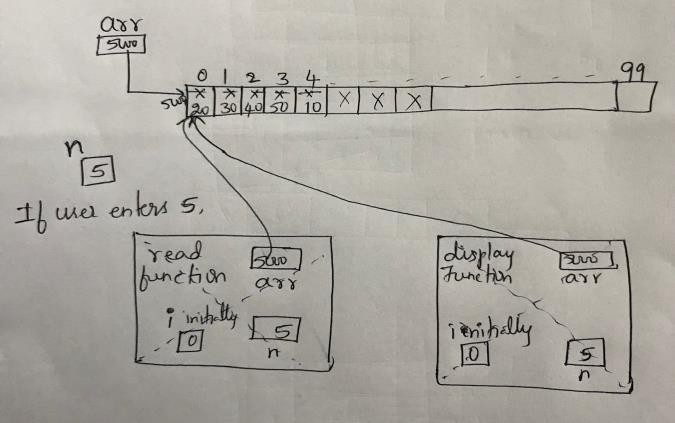You can write this code using client and server having a clear line between interface and implementation. Use make files and make command for the same.

Let us try to pass array as an argument and create one more array using this array in the main function

//int a[] read(int arr[],int n); //return type cannot be array

void display(int *arr, int n);

int main()

{ int arr; int n;

printf("Enter the number of elements you want to store\n");

scanf("%d",&n);

printf("enter %d elements\n",n);

// int b[] = read(arr,n);     // this throws compile-time Error. Cannot return an array

// So change b to pointer and return type of function to pointer.

printf("entered elements in arr\n");

display(arr,n);

printf("Elements in b\n");

display(arr,n);

return 0;

}

int* read(int *arr, int n) // observe return type int*

{ int i = 0;

while(i<n)

{ scanf("%d",&arr[i]);

i++;

}

return arr;

}

void display(int *arr, int n)

{ int i = 0;

while(i<n)

{ printf("%d\t",arr[i]);

i++;

}

}

### 3.9 Recursion

A function may call itself directly or indirectly. The function calling itself with the termination/stop/base condition is known as recursion. Recursion is used to solve various problems by dividing it into smaller problems. We can opt if and recursion instead of looping statements. In recursion, we try to express the solution to a problem in terms of the problem itself, but of a smaller size.

Consider the below code.

int main()

{

printf("Hello everyone\n");

main();

return 0;

}

Execution starts from main() function. Hello everyone gets printed. Then call to main() function. Again, Hello everyone gets printed and these repeats. we have not defined any condition for the program to exit, results in Infinite Recursion. In order to prevent infinite recursive calls, we need to define proper base condition in a recursive function.

Let us write Iterative and Recursive functions to find the Factorial of a given number. client.c is given as below.

int fact(int n);

int main()

{ int n = 6;

printf("factorial of %d is %d\n",fact(n));

}

Iterative Implementation:

int fact(int n)

{ int result = 1; int i;

for (i = 1; i<=n; i++)

{

result = result * i;

}

return result;

}

Recursive Implementation:

Logic:

If n is 0 or n is 1, result is 1

Else, result is n*(n-1)!

int fact(int n)

{ if (n==0)

return 1;

else

{ return n*fact(n-1); }

}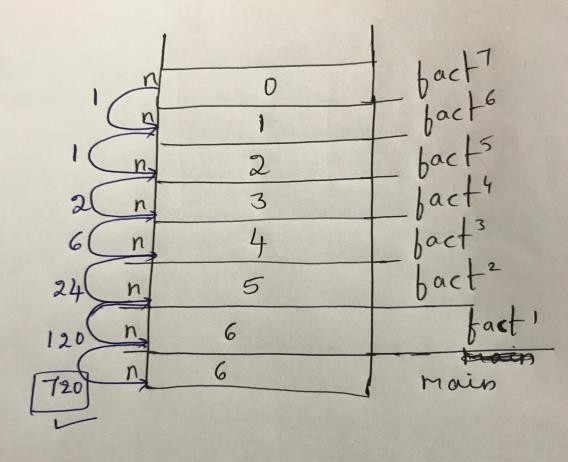Let us start tracing the given recursive function

Example 1: What this function does?

int what1(int n){

if (n == 0)

{ return 0; }

else

{ return (n % 2) + 10 * what1(n / 2); }

}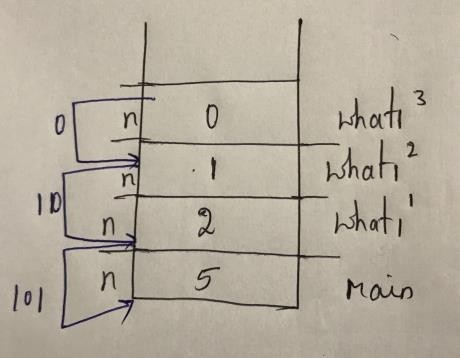// if n is 5, refer to the diagram.

This code prints the given number in binary.

Example 2:

Version 1:

int what2_v1(int n)

{ if (n == 0)

return 0;

else

return (n & 1) + what2_v1(n >> 1);

}

//Finds the number of 1s in the binary representation of the number

Version 2:

int what2_v2(int n)

{

if(!n)

{ return 0; } else if(!(n % 2))

{

else return what2_v2(n / 2); }

{ return 1+what2_v2(n / 2); }

}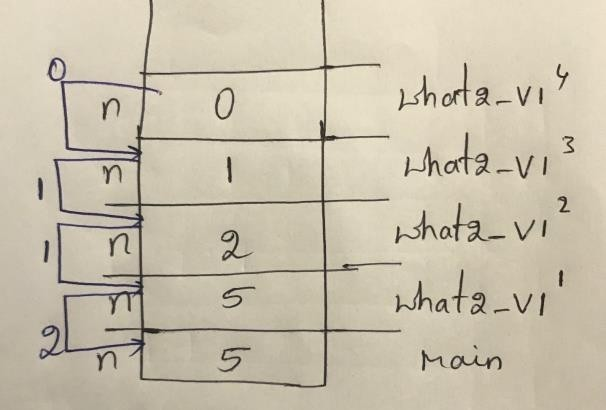Try to trace below with diagram.

Example 3:

Version 1:

int what3_v1(int b,int p)

{

int result=1;

if(p==0)

return result;

result=b*(what3_v1(b,p-1));

}

Version 2:

int what3_v2(int b, int p)

if(!p)

return 1;

else if(!(p % 2))

return what3_v2(b*b, p/2);

else

return b*what3_v2(b*b,p/2);

Example 3 Finds b to the power p

Example 4:

int what4(int n)

{

if (n>0)

return n + what4(n - 1);

else if (n==0)

return 0;

else return -1;

If the given number is n, Finds n+(n-1)+(n-2)+ … +0

If n is -ve, returns -1.

Few problems to Code:

Recursive function to reverse a string

Recursive function to print from 1 to n in reverse order

Find the addition, subtraction and multiplication of two numbers using recursion. Write separate            recursive functions to perform these.

Find all combinations of words formed from Mobile Keypad.

Find the given string is palindrome or not using Recursion.

Find all permutations of a given string using Recursion

## Chapter 4: String in C

A string in C is an array of characters and terminated with a special character ‘\0’ or NULL. ASCII value of NULL character is 0. Each character occupies 1 byte of memory. There is NO datatype available to represent a string in C.

char ch = 'x' ; // character constant in single quote. always of 1 byte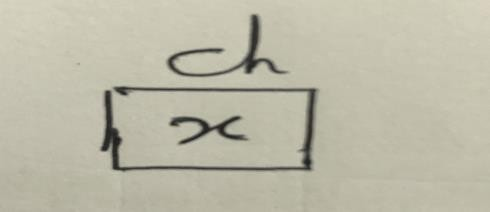char ch[] = "x" ; // String constant. always in double quotes. Terminated with '\0'. Always 1 byte more when specified between “ and ” in initialization.